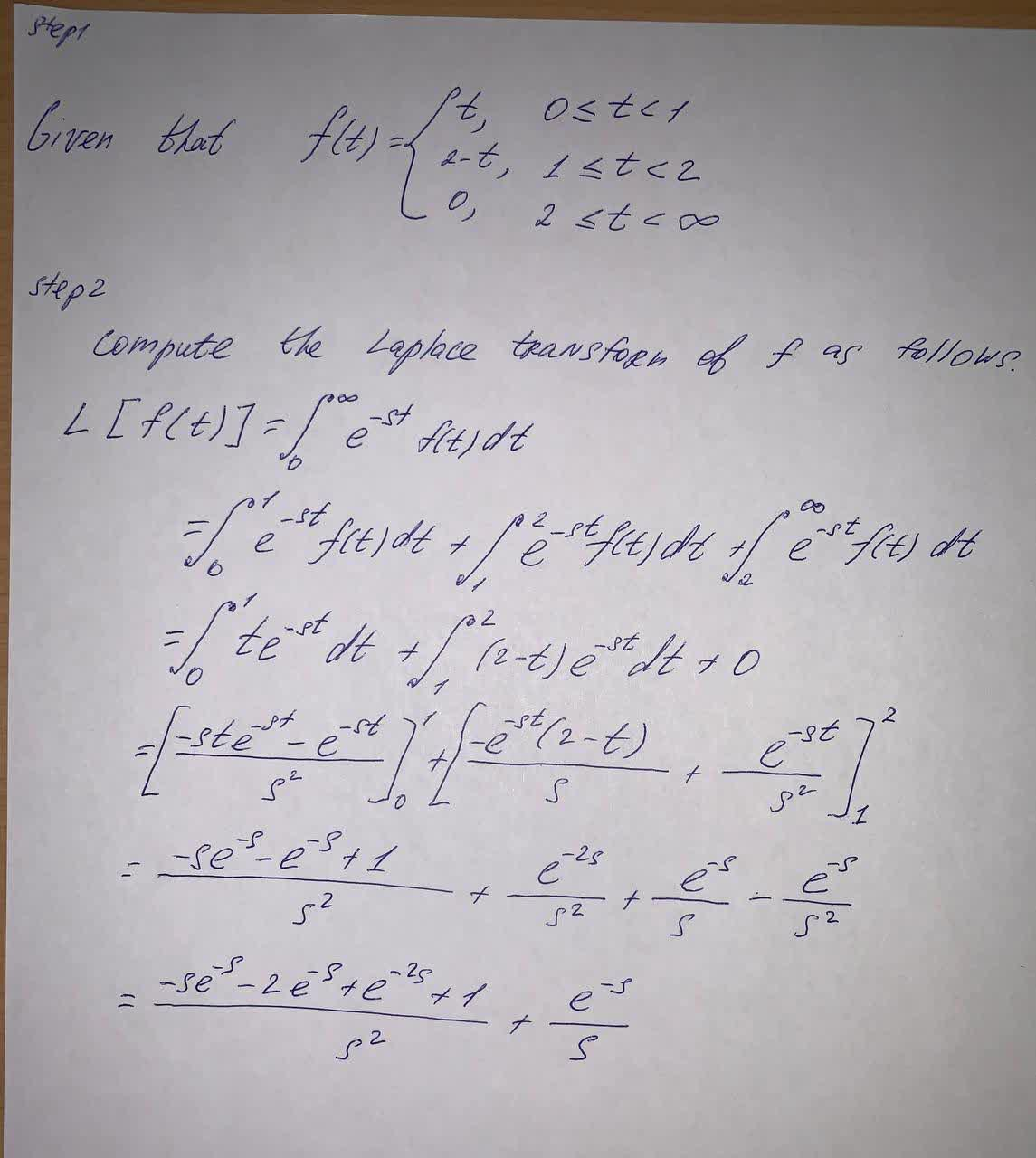# find the Laplace transform of the given function. f(t)={(t, 0\leq t <1),(2-t, 1\leq t <2),(0, 2\leq t < \infty):}Cem Hayes 2021-09-17 Answered

find the Laplace transform of the given function.
$f\left(t\right)=\left\{\begin{array}{ll}t,& 0\le t<1\\ 2-t,& 1\le t<2\\ 0,& 2\le t<\mathrm{\infty }\end{array}$

You can still ask an expert for help

## Want to know more about Laplace transform?

• Live experts 24/7
• Questions are typically answered in as fast as 30 minutes
• Personalized clear answers

Solve your problem for the price of one coffee

• Math expert for every subject
• Pay only if we can solve itAnonym
Given function isWe have step-by-step solutions for your answer!